## 9-1 嚙踝蕭嚙踝蕭嚙踝蕭嚙踝蕭嚙踝蕭嚙踝蕭嚙踝蕭嚙?

• 基於多項式的內插法
• 基於FFT（Fast Fourier Transform，快速傅立葉轉換）的內插法

MATLAB 最常用的一維內插指令是 interp1，其原理是利用多項式來進行內插運算。interp1 的使用語法為

yi = interp1(x, y, xi, method)

• 'nearest'：鄰近點內插法
• 'linear'：線性內插法
• 'spline'：片段式的三次 Spline 內插法
• 'pchip'：保持形狀的片段式三次內插
• 'cubic'：和'pchip' 一樣
• 'v5cubic'：MATLAB 5 所用的三次內插法

Example 1: 09-內插法/interp101.mx = 0:1:4*pi; y = sin(x).*exp(-x/5); xi = 0:0.1:4*pi; y1 = interp1(x, y, xi, 'nearest'); y2 = interp1(x, y, xi, 'linear'); y3 = interp1(x, y, xi, 'pchip'); y4 = interp1(x, y, xi, 'spline'); plot(x, y, 'o', xi, y1, xi, y2, xi, y3, xi, y4); legend('Original', 'Nearest', 'Linear', 'Pchip', 'Spline');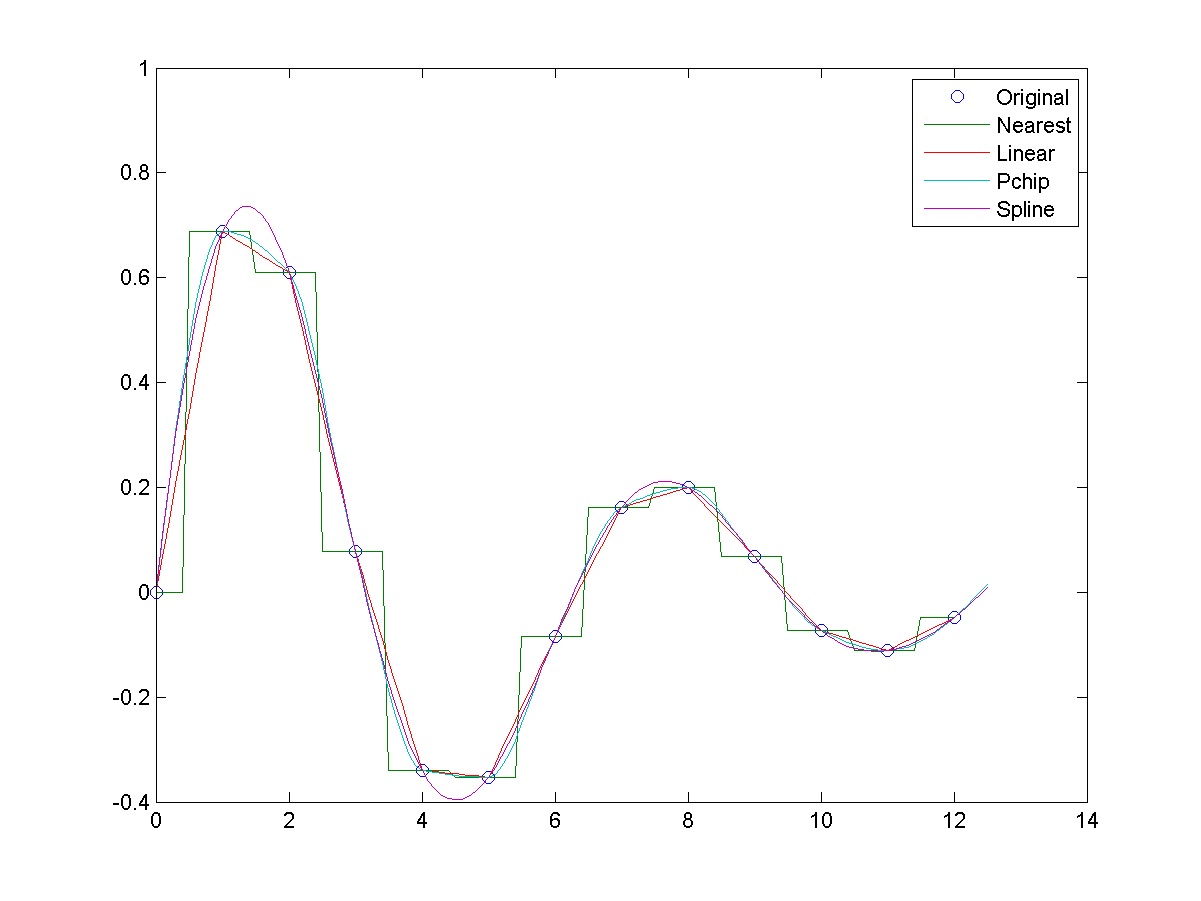Nearest Linear Cubic Cubic Spline

Hint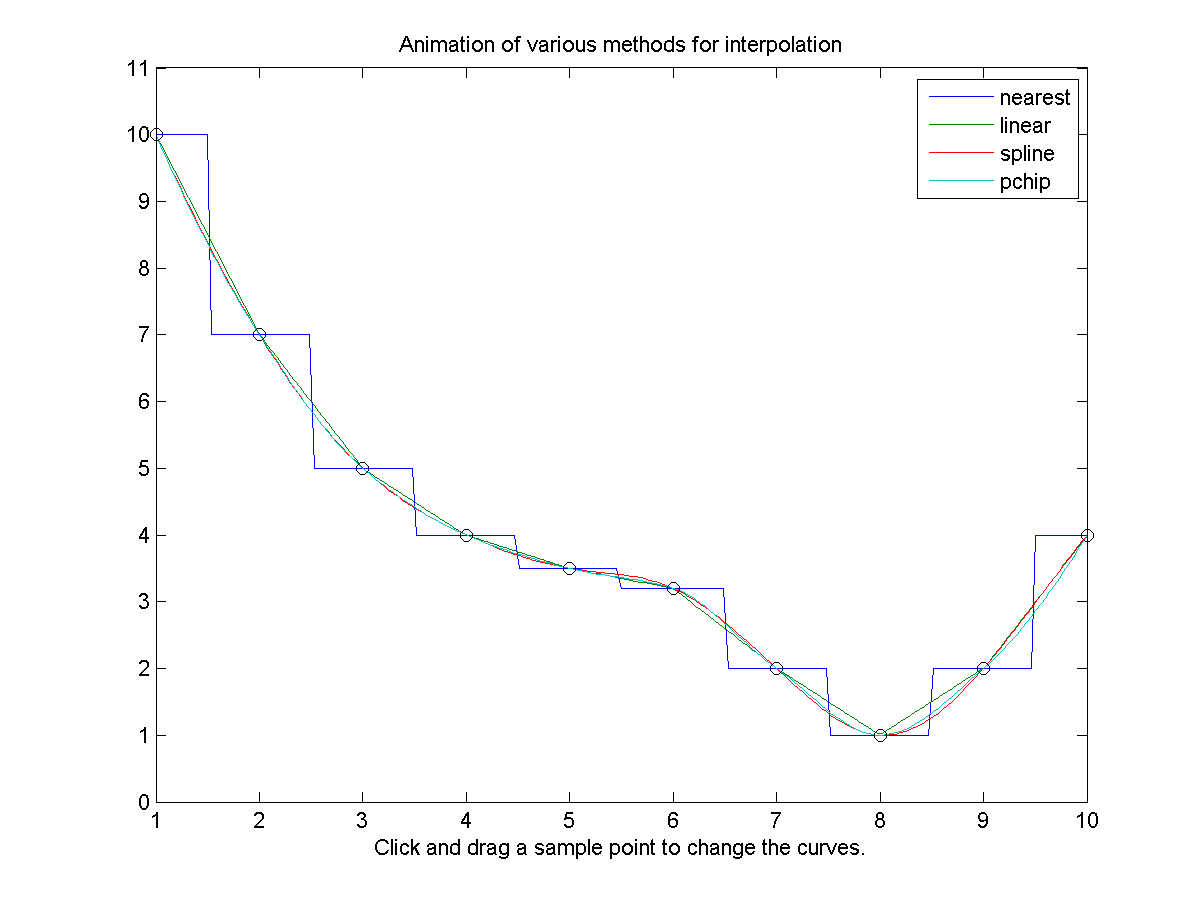y = interpft(yi, n)

Example 2: 09-內插法/interpft01.mn = 11; % Number of the original data points factor = 4; % Increase the data by this factor x = linspace(0, 2*pi, n); y = sin(x).*exp(-x/5); xi = (0:factor*n-1)*(x(2)-x(1))/factor; yi = interpft(y, factor*n); plot(x, y, 'ro', xi, yi, '.-'); legend('Original', 'Curve by interpft');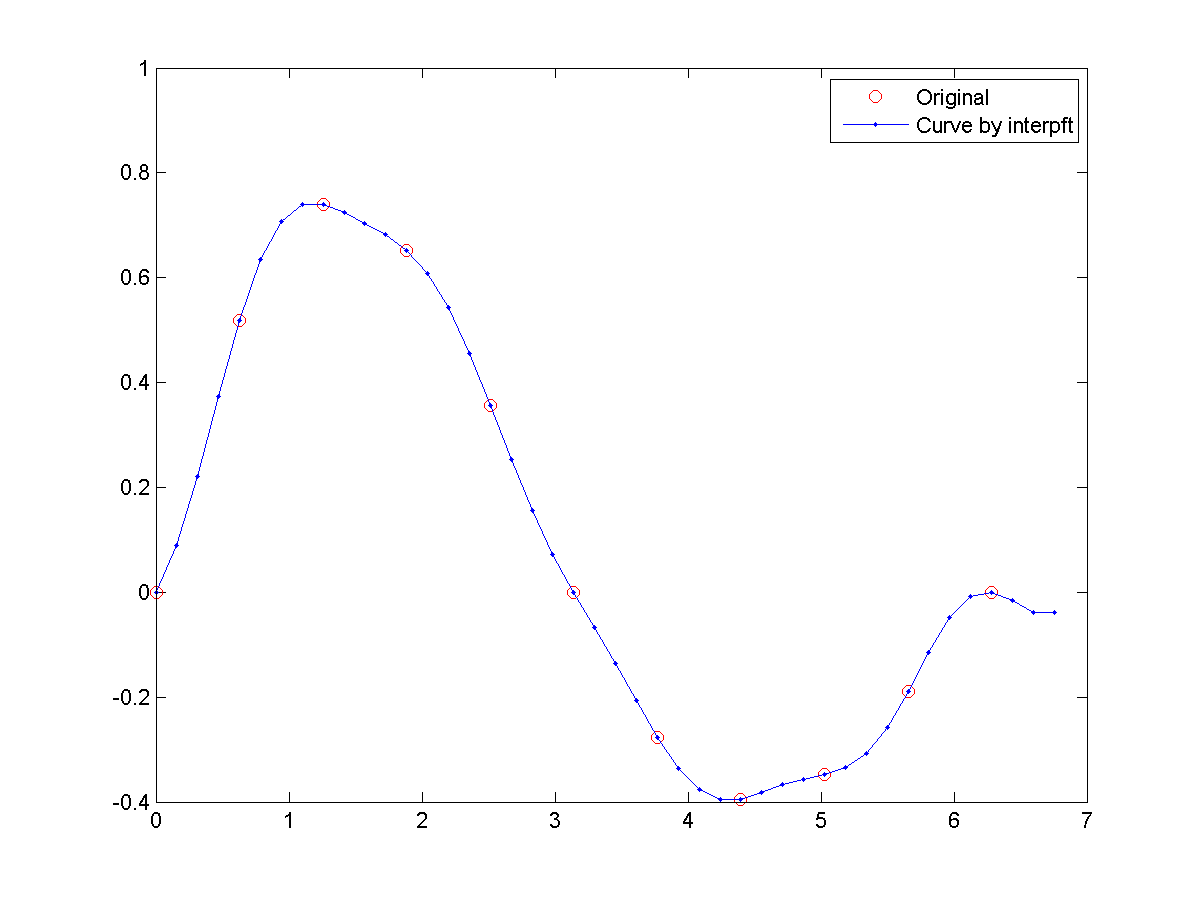Hint

• X座標對其索引（Index，或稱指標）的內插
• Y座標對其索引（Index，或稱指標）的內插

Example 3: 09-內插法/interp102.mx = [0 2 4 3 1 2 1]; y = [4 1 2 4 5 2 0]; index = 1:length(x); index2 = linspace(1, length(x), 101); x2 = interp1(index, x, index2, 'spline'); y2 = interp1(index, y, index2, 'spline'); plot(x, y, 'o', x2, y2, '-'); legend('Origianl data', 'Interpolated data');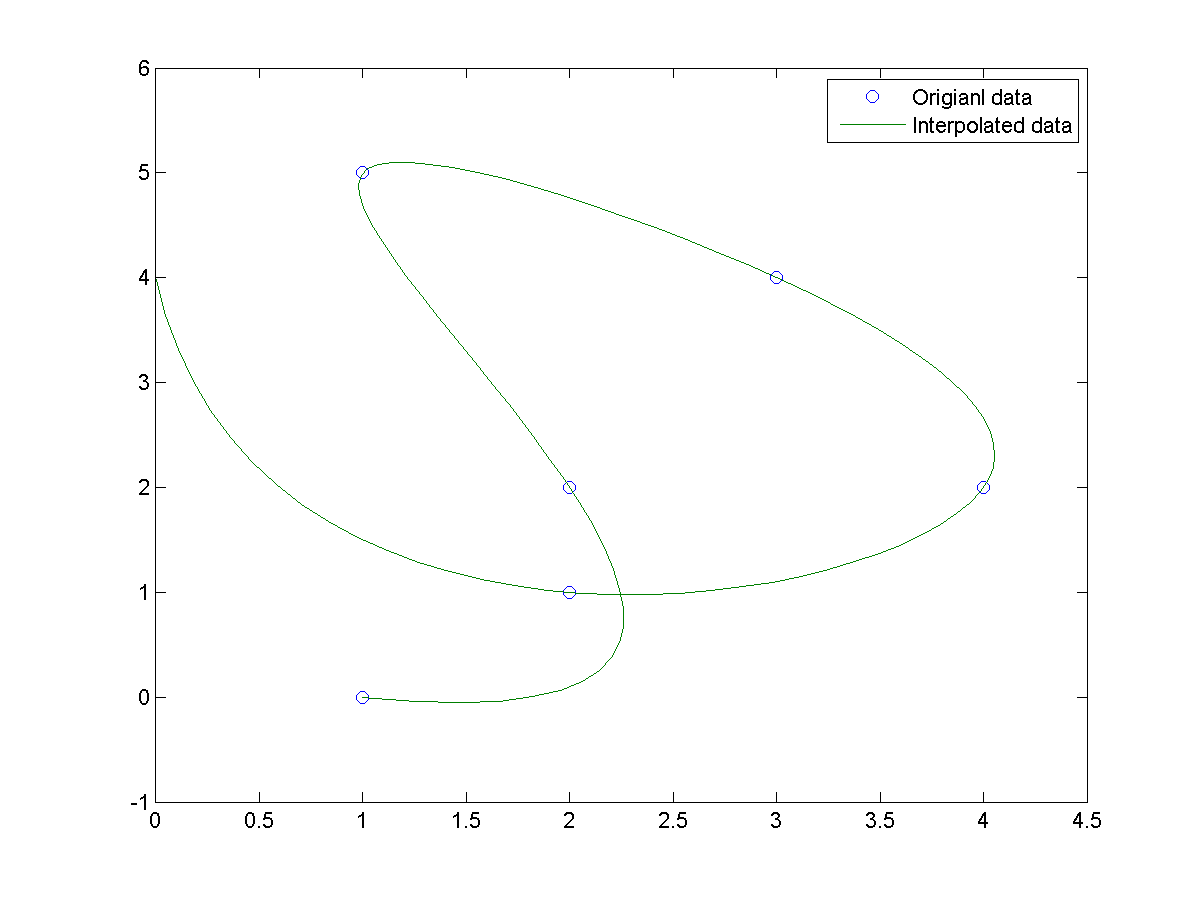Example 4: 09-內插法/interp103.mx = [0 2 4 3 1 2 1]; y = [4 1 2 4 5 2 0]; index = 1:length(x); index2 = linspace(1, length(x), 101); x2 = interp1(index, x, index2, 'nearest'); y2 = interp1(index, y, index2, 'nearest'); x3 = interp1(index, x, index2, 'linear'); y3 = interp1(index, y, index2, 'linear'); x4 = interp1(index, x, index2, 'pchip'); y4 = interp1(index, y, index2, 'pchip'); x5 = interp1(index, x, index2, 'spline'); y5 = interp1(index, y, index2, 'spline'); plot(x, y, 'o', x2, y2, '-'); plot(x, y , 'o', x2 ,y2, x3 ,y3 ,x4 ,y4, x5, y5); legend('Original', 'Nearest', 'Linear', 'Pchip', 'Spline');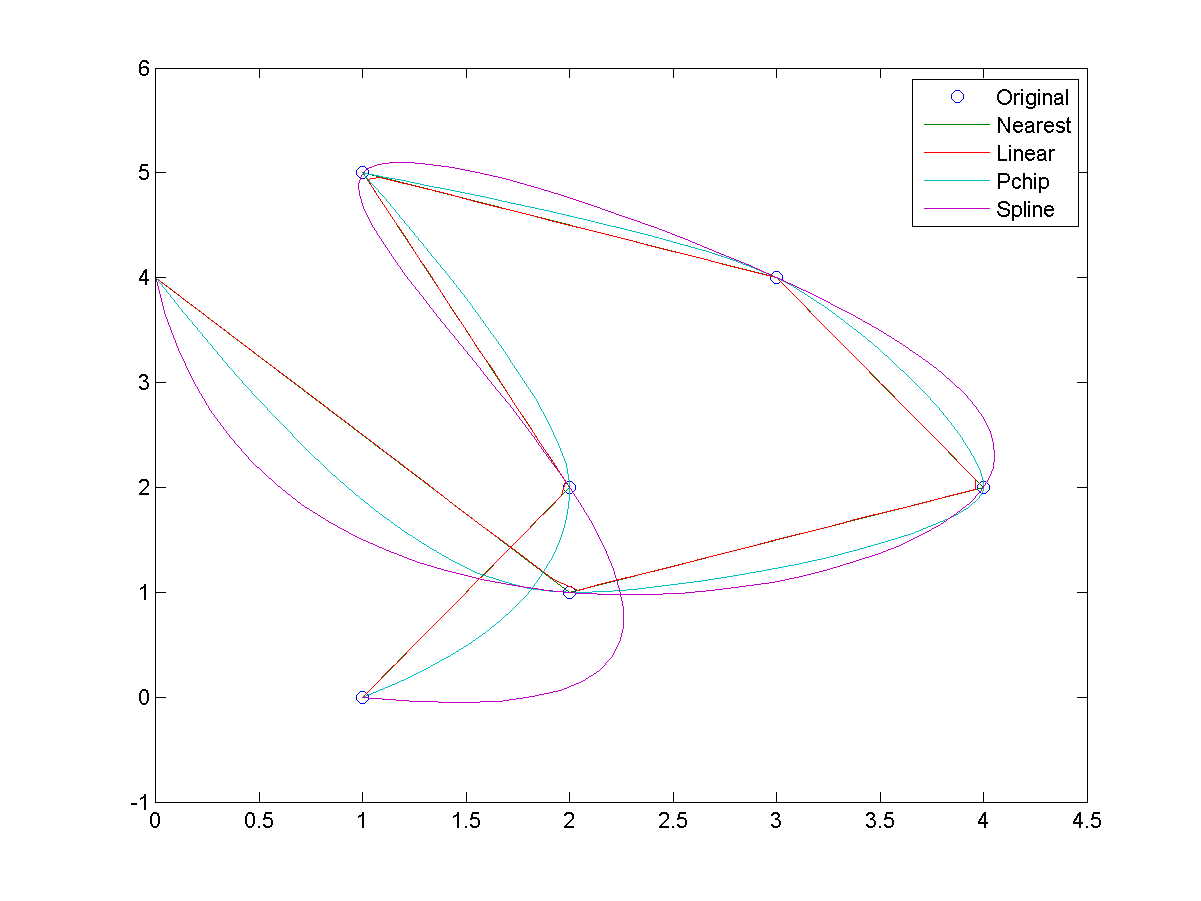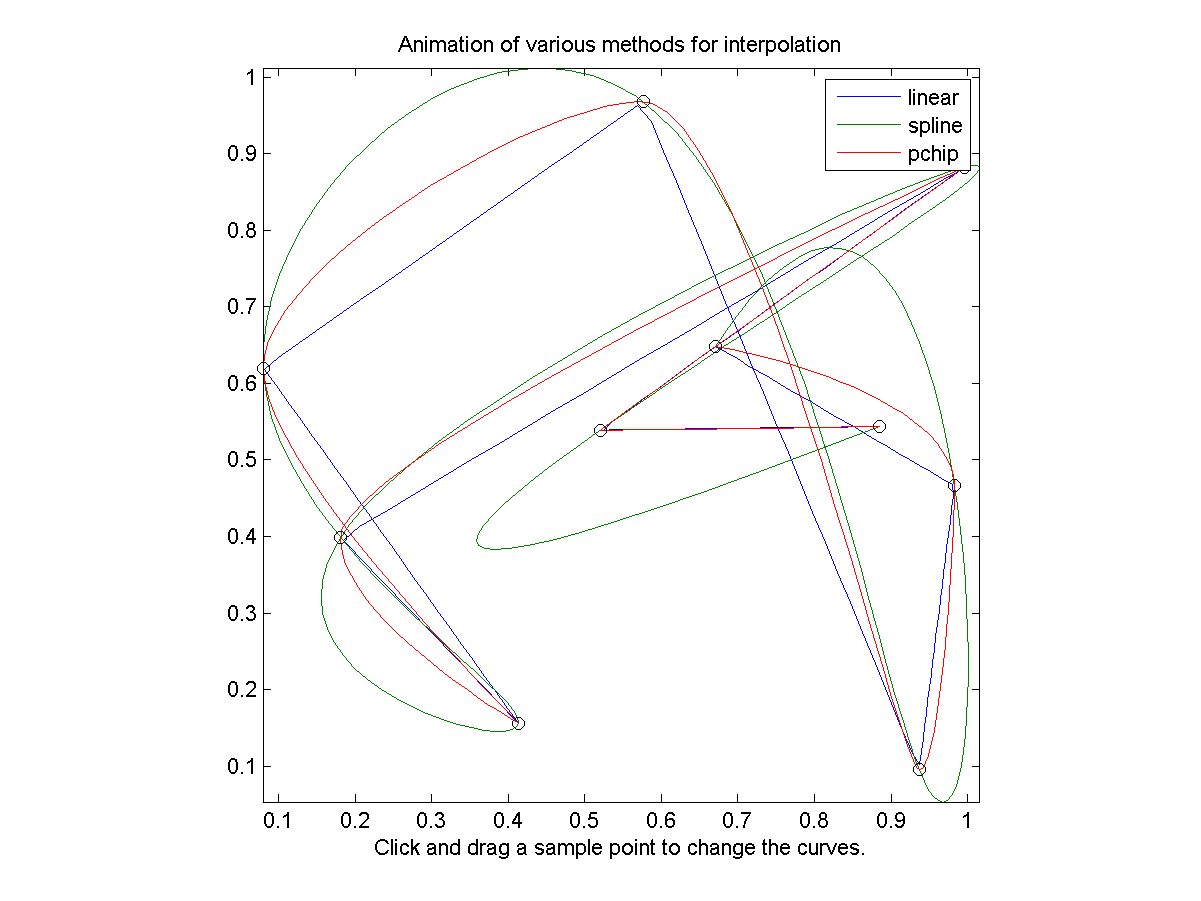MATLAB程式設計：進階篇